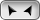## Welcome to OnlineConversion.com

Acceleration Conversion

 Convert what quantity? From: centigal centimeter/square second decigal decimeter/square second dekameter/square second foot/square second g-unit (G) gal galileo gn grav hectometer/square second inch/square second kilometer/hour second kilometer/square second meter/square second mile/hour minute mile/hour second mile/square second milligal millimeter/square second To: centigal centimeter/square second decigal decimeter/square second dekameter/square second foot/square second g-unit (G) gal galileo gn grav hectometer/square second inch/square second kilometer/hour second kilometer/square second meter/square second mile/hour minute mile/hour second mile/square second milligal millimeter/square secondInformation:
- The SI standard unit for acceleration is meters/square second.
- m/s2 is meters/square second.

### BookMark Us

It may come in handy.

Are you bored?

Try the Fun Stuff